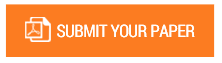Numerical Solutions of the Work Done on Finite Order-Preserving Injective Partial Transformation Semigroup

Authors : Rotimi Kehinde; Abdulazeez O Habib

Volume/Issue : Volume 5 - 2020, Issue 9 - September

Scribd : https://bit.ly/3kkJrfD

DOI : 10.38124/IJISRT20SEP119

In this research work, we have used an effective methodology to obtain formulas for calculating the total work done and consequently the average work done and the power of the transformation by elements of finite order-preserving injective partial transformation semigroup. The generalized formulas was applied to obtain the numerical solutions and results tabulated. We equally plot graphs to illustrate the nature of the total work done and the average work done by elements of the finite order-preserving partial transformation semigroup. Results obtained showed that moving the elements from domain to co-domain involve allot of work to be done on a numerical scale

Keywords : Partial Transformation, Semigroup, Total Work Done, Average Work Done, Power, Injective

#### CALL FOR PAPERS

Paper Submission Last Date
31 - May - 2022